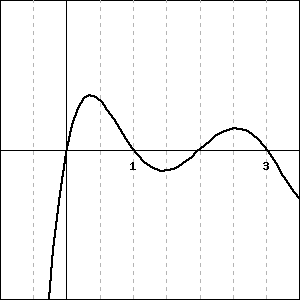Consider the function $y = f(x)$ graphed below.Give the $x$-coordinate of a point where:
A. the derivative of the function is negative: $x =$
B. the value of the function is negative: $x =$
C. the derivative of the function is smallest (most negative): $x =$
D. the derivative of the function is zero: $x =$
E. the derivative of the function is approximately the same as the derivative at $x = 3.25$ (be sure that you give a point that is distinct from $x = 3.25$!): $x =$